#### Vol. 37, No. 3, 1971

 Download this articleFor screen For printingRecent Issues Vol. 325: 1  2 Vol. 324: 1  2 Vol. 323: 1  2 Vol. 322: 1  2 Vol. 321: 1  2 Vol. 320: 1  2 Vol. 319: 1  2 Vol. 318: 1  2Online Archive Volume: Issue:The Journal Subscriptions Editorial Board Officers Contacts Submission Guidelines Submission Form Policies for Authors ISSN: 1945-5844 (e-only) ISSN: 0030-8730 (print) Special Issues Author Index To Appear Other MSP Journals
An estimate for Wiener integrals connected with squared error in a Fourier series approximation

### Frederick Stern

Vol. 37 (1971), No. 3, 813–815
##### Abstract

If a function x(σ),0 σ t, is in Lipα,0 < α < 1,x(0) = 0 and if ck(k = 0,1,2,) are its Fourier coefficients with respect to the functions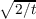sin[π(k +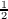)σ∕t], then it is known [1, pp. 171–172] that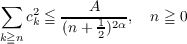(1)

where A is a positive number not depending on n. We will show a connection between this estimate and an estimate for Wiener integrals. Let Ew{ } denote expectation on a Wiener process, that is, a Gaussian process with mean function zero, covariance function min(σ,τ),0 σ,τ t and sample functions z(σ) with z(0) = 0.

Theorem: Let x(σ) be in C[0,t] and let ck be the Fourier coefficients of x(σ) with respect to the normalized eigenfunctions associated with min(σ,τ). That is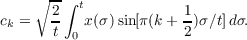Let 0 < α < 1. Then estimate (1) is a necessary and sufficient condition for the estimate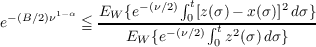(2)

for all positive ν, where B is a positive number not depending on ν.

Primary: 42A16
Secondary: 28A40
##### Milestones